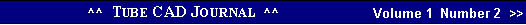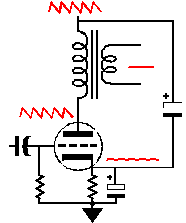capacitor to ensure that the power bandwidth would extend to the lowest frequencies. The value of this capacitor is set by the following formula:          Ck = 1 / (2pFRk)  or  Ck = 159155 / F / Rkwhere F equals the lowest frequency to be reproduced, usually 2 to 20 Hz. If we set the value to 30 µF, the cutoff frequency will be 2.65 Hz -- plenty low.     Interjecting the correct amount of power supply noise at the cathode will require knowing the gain this tube realizes in the circuit. Let us set the value to 3; in other words, 10 volts of signal at the cathode will result in 30 volts of swing at the plate. We must now add one to the gain to determine the amount of gain the tube will realize if driven from its cathode rather than its grid.     The gain increase results from the cathode being further from the plate than the grid. This increased distance results in a greater effective amplification factor for the cathode. And since the distance between grid and cathode sets the denominator for the distance from the grid to the plate, when evaluating the mu of a triode, the cathode would have to be one denominator unit further away from the plate than the grid; therefore, it must have plus one more mu than the grid. This increase in mu is reflected in the formula for gain of a Grounded Grid amplifier:       G = (mu + 1)Rl / (rp + Rl) versus the gain for a Grounded Cathode amplifier:       G = mu·Rl / (rp + Rl).    Having set the gain from the cathode to approximately 4, we now need to interject one forth the power supply noise into the cathode. Since the cathode was bypassed with a 30 µF capacitor, we must calculate the value of a capacitor to connect from the cathode to the B+ connection of the output
 transformer. Since we want a quarter of the power supply noise to develop across the bypass capacitor, the value of the extra capacitor would have to be 3 times smaller:       Ratio = C / ( C + Ck).In this case, the capacitor value would be 10 µF. As this capacitor will see virtually all of the power supply voltage developed across its plates, it must be voltage rated as such. Thiscapacitor also serves to augment or maybe supplant the existing power supply capacitor. Do not forget that capacitors in series do not add together as do resistors; instead, the values must be paralleled to calculate the resulting capacitance:        C  = C1C2 / (C1 + C2).Thus the resulting capacitance in this example would be only 7.5 µF, not 40 µF.Conclusion    This technique lowers the amount of power supply noise from output without having reduced the amount of power supply noise. Reducing this noise at the source could only improve the benefits from this technique. // JRBpg. 4